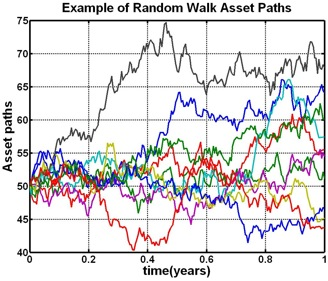# How to do simulation in Dynare?

I am not talking about a one period shock, and then you observe the IRF, I am talking about simulating time series for endogenous variables given that we know the distribution of exogenous variable.

1. For example, if I have the TFP equation (AR 1 process), and I know the rho and I know the variance of the error, can we generate a time series of TFP in Dynare?

2. If we can generate exogenous time series like in 1, can we also generate time series for endogenous variables?

The reason why I am asking is, I would like to see where a variable would end up after a few periods if random shocks from a known dist happen during those period.

1. specify the distribution of the shock which also determines the distribution of the exogenous variable

var ea; stderr 0.01;

1. Simulate the endogenous variables. Here, Y and C.

stoch_simul(periods=1000) Y C

Check more options for these commands from the manual.

But that is not what I wanted. What you told me, is what would happen to Y when there is a one time shock to ea.

What I want is; what would Y look like when there is a shock every single period for 200 periods. The size of this shock is drawn from a distribution. Basically I want a computer generated path from Y, according to the specified distribution of the shocks.

Something like this, but for the endogenous variables.``````stoch_simul(periods=1000)...
``````

will draw random shocks for 1000 periods and compute the simulation. If you plot the results in `oo_.endo_simul` you will get a graph similar to the one you show.

Exactly. And if you want more than 1 sample path, use the `simul_replic`-option. See e.g.

This is the solution.

I thought that those variables are the IRF, until I plotted them.

`oo_.endo_simul` contains the IRF when you don’t use option `periods` in the `stoch_simul` command

Thank you so much.

There are just too many options etc in Dynare, that I never explored. I really thought it could only show IRF.

Merci, les equipe Dynare.

One final question, I hope; is there a way to specify the initial value of the simulation? I.e. if I want the sim to start from specific points.

See the Dynare manual on `histval`.